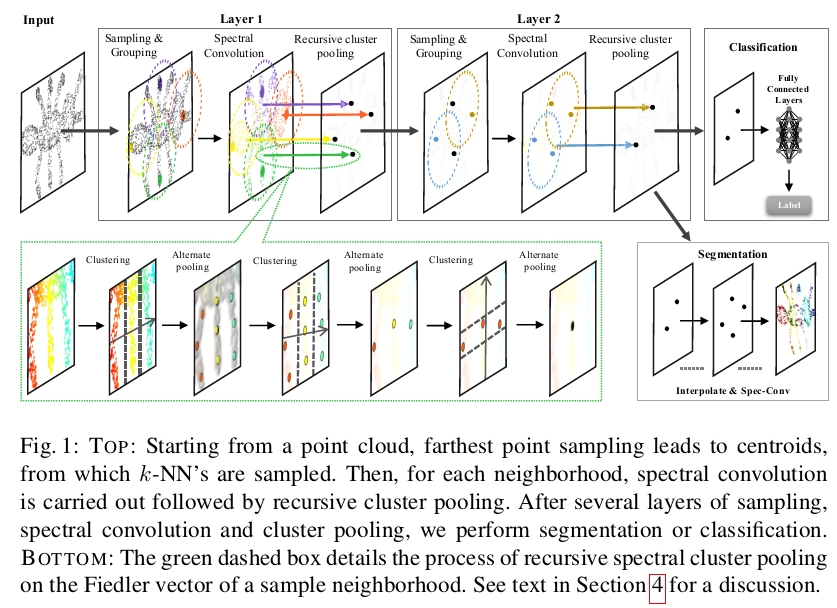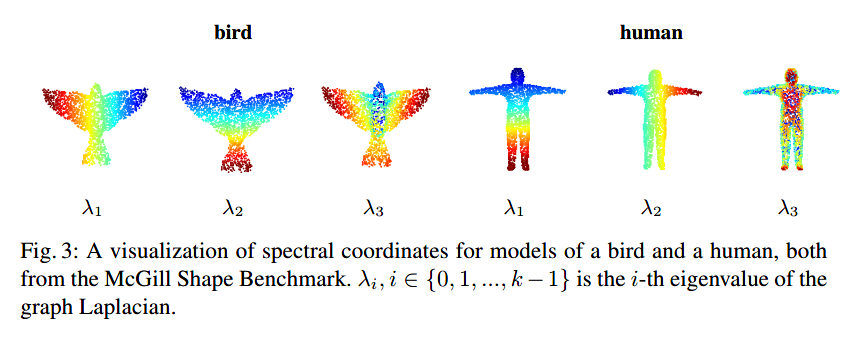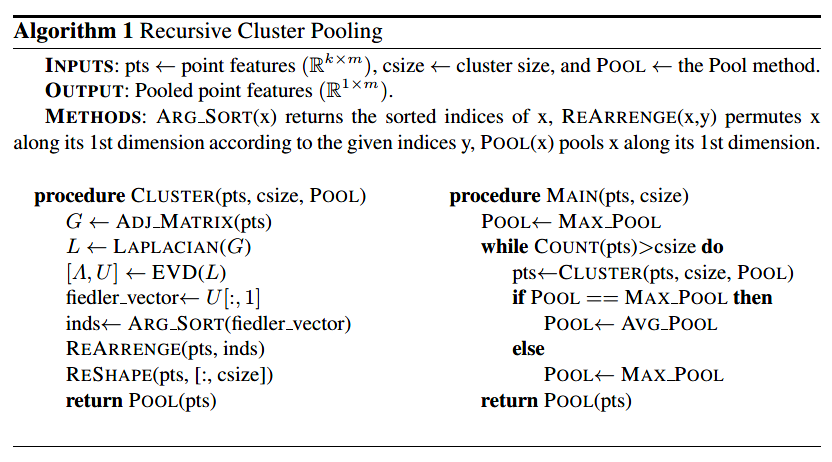# 译：Local Spectral Graph Convolution for Point Set Feature Learning-用于点集特征学习的局部谱图卷积

## 1 Introduction• 在点集特征学习中使用局部谱图卷积以在每个点的邻域中结合结构信息。
• 局部频谱图卷积层的实现，其不需要离线计算并且可以端到端方式训练。我们在运行时动态构建图，并动态计算拉普拉斯算子和池化层次结构。
• 使用新颖有效的图池化策略，通过递归聚类谱坐标来聚合图节点处的特征。

## 2 Challenges in point set feature learning

Pointnet ++框架中特征学习的局限性在于，一个点的k个最近邻（k-NN）的特征是以孤立的方式学习的。设$h$表示深层网络中任意隐藏层的输出，通常是多层感知器。在Pointnet ++中，$k$-NN中每个点的各个特征都是用$h(x_i),i \in 1,2,...,k$实现的。不幸的是，这个隐藏层函数不模拟k-NN中各点之间的联合关系。一个联合学习来自k-NN中的所有点的特征的卷积核将捕获与点的几何布局相关的拓扑信息，以及与输入点样本本身相关的特征，例如颜色，纹理或其他属性。在下一节中，我们将扩展诸如Pointnet ++框架之类的方法，以通过使用局部图卷积来实现这一目标，不过是在谱域中。

Pointnet ++的另一个限制是$k$-NN的集合激活函数是通过跨每个点的隐藏层输出的最大池化来实现的，也就是说

$f(x_1,x_2,...,x_k)=max_{i \in 1,...,k}h(x_i)$

$f(x_1,x_2,...,x_k)=\bigoplus(h_1,h_2,...,h_k)$## 3 Graph Convolution 图卷积

$h=X*g$

$\tilde h=\tilde X \bigodot \tilde g$

### 3.2 Graph Fourier Transform 图傅里叶变换

$L=I-D^{1/2}WD^{1/2}$

$U=[u_0,u_1,...,u_{k-1}]\in R^{k\times k}$

$\Lambda=diag([\lambda_0,\lambda_1,...,\lambda_{k-1}])\in R^{k\times k}$

### 3.3 Spectral Filtering 谱滤波

$x*g=U((U^T x)\bigodot (U^Tg))$

$y=\tilde g_\theta(L)X=\tilde g_\theta (U\Lambda U^T)X=U\tilde g_\theta (\Lambda)\tilde X$

### 3.4 Implementation of Spectral Filtering 谱滤波的实现

1. 频谱调制 输出$P = G\tilde X$，对角矩阵$G$是非参数化的核$\tilde g_\theta(\Lambda)$。$G$的$k$个对角线条目都是非参数化傅立叶核公式中的自由参数。

2. 特征过滤 它将输入维度从$m$扩展到$m\prime$。该步骤的输出是特征矩阵$Q\in R^{k\times m\prime}$。条目$q_{k,i}$是第$k$个点的第$i$个输出特征并且由$q_{k,i}=\sum_{j=1}^m p_{k,j}w_{j,i}$这里$p_{k,j}$是对应于在前一步骤中定义$P$的第k个点的第$j$条输入特征，$w_{j,i}$是第$j$个输出特征和第$i$个输入特征之间的滤波器系数。该步骤可以由$Q = P W$表示，其中$W$是可学习滤波器的参数矩阵。步骤1和2中的过滤操作可以概括为

$Q=(G\tilde X)W$

3. 傅里叶逆变换 它通过$y = UQ$在空间图信号域中提供卷积输出。

## 4 Pooling on Local $k$-NN Graph 局部$k$-NN图的池化### 4.2 Clustering, Pooling and Recurrences 聚类，池化和重现## 译者致谢

posted @ 2018-09-13 22:58  ElliottZheng  阅读(...)  评论(...编辑  收藏